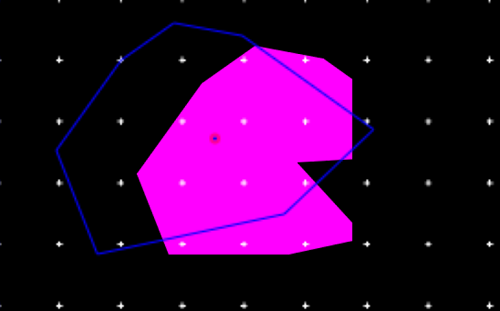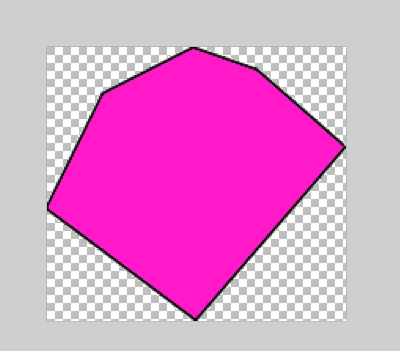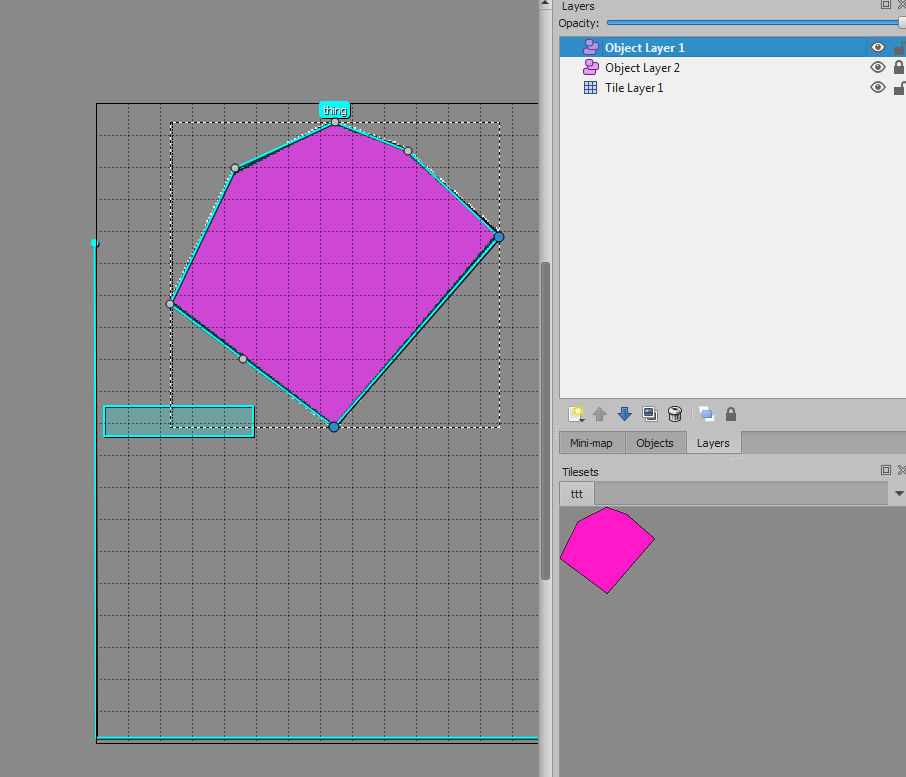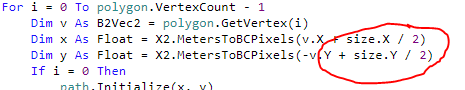Games Filling of polygon and convert to ScaledBitmap not drawing

Discussion in 'Game Development' started by Gunther, Nov 27, 2018.

1. Hello,

I'm trying to put the following code in the Game.Initiatization procedure with the background to create from the shape's fixture of the body (here bw) a polygon and use this polygon to create a bitmap to be used as the preloaded graphic for the GraphicCache.

The vertics are less then 8 pcs.

So, not the approach to use it in the Game.Tick.

When changed to the garadient filled rect it works almost as expected, but this is a rect which is seen on the screen on the right location over the shape.

But as version using the brush it looks not as expected.

Any suggestion? B4J-Testproject attached.Code:
`'                Dim PolygonPath As BCPath                 Dim polygon As B2PolygonShape =  bw.Body.FirstFixture.Shape                ' Starting Point of Polygone                Dim PrevVertex As B2Vec2 = bw.body.GetWorldPoint(polygon.GetVertex(0))                PrevVertex = X2.WorldPointToMainBC(PrevVertex.X, PrevVertex.Y)                PolygonPath.Initialize(PrevVertex.X, PrevVertex.Y)                ' filling path + transfer of World points of shape to BCMain Points                For i = 1 To polygon.VertexCount                    Dim vertex As B2Vec2 = bw.body.GetWorldPoint(polygon.GetVertex(i))                    vertex = X2.WorldPointToMainBC(vertex.X, vertex.Y)                    PolygonPath.LineTo(vertex.X, vertex.Y)                    PrevVertex = vertex'                    Log(vertex)                Next                ' close of polygon                PolygonPath.LineTo(PrevVertex.X, PrevVertex.Y)                '                Dim size As B2Vec2 = X2.GetShapeWidthAndHeight(template.FixtureDef.Shape)                Dim rect As B4XRect                rect.Initialize(0, 0, X2.MetersToBCPixels(size.X), X2.MetersToBCPixels(size.Y))                Dim bc As BitmapCreator = X2.GraphicCache.GetBitmapCreator(rect.Height)                '' ************************'                ' for Garadient filling of the rect'                bc.FillGradient(Array As Int(Rnd(0xff000000, -1), Rnd(0xff000000, -1)), rect, "TL_BR")'                Dim Brush As BCBrush = bc.CreateBrushFromBitmapCreator(bc)' ************************                ' for the polygone                Dim Brush As BCBrush = bc.CreateBrushFromColor(xui.Color_Magenta)                '                bc.DrawPath2(PolygonPath, Brush, True, 10)' ************************'                Dim sb As X2ScaledBitmap                sb.Scale = 1                sb.Bmp = bc.Bitmap.Crop(0, 0, rect.Right, rect.Bottom)                 X2.GraphicCache.PutGraphic2(template.CustomProps.Get("graphic name"), Array(sb), True, 1)`

Attached Files:

• TestProject.zip
File size:
8.2 KB
Views:
41
Last edited: Nov 27, 2018
2. all moduls are missing. please include them in your zip file

3. Here zipped from IDE.

Well, may be it has to do with the scaling of Tiled/MainBC/TargetViewWidthInMeters/TileWidthMeters etc.

Attached Files:

• TestProject2.zip
File size:
27.2 KB
Views:
40
4. the pink one is not a convex vector maybe that's the problem?

5. Why is this hard work needed? Why not create a bitmap file and set the 'graphic file 1' property?

6. Step 1: Create the polygon image however you like:
Paint .Net:Make sure that there is no extra empty space left.

Step 2: Add the image to Tiled as a tileset made of a collection of images.

Step 3: Create a new object layer that is only used for Tile objects. They will never be loaded. Add a Tile object and resize it as you like.
You can now lock this layer.

Step 4:

Modify the polygon object to match the image:That's it.

Code:
`For Each template As X2TileObjectTemplate In ol.ObjectsById.Values       If template.Name <> "hinge" Then           Dim bw As X2BodyWrapper = TileMap.CreateObject(template)       End If   Next`andymc and sorex like this.
7. Thanks for the replies.

I know that the normal way is to add it via the graphic file and adding it via Tiled as written.
And as you may know this is always as much as possible reduced test thing of a much bigger one to see what is possible and where.

I was just thinking if it is easy to fill a polygon and the polygon is from a fixture. Just to avoild always to run the app in DebugDraw Mode to see the fixtures/bodies while creation of the app.

In the Tick Evend it is easy to draw something in between the fixtures by using the Future Drawtasks, e.g. some times you have two bodies and if they are moveing realtivly to each other gap popping up. See Joints Example between the bridge parts.

Or here they have an outline but this black line don't go across the knee.Last edited: Nov 27, 2018
8. I'm not sure that you understood correctly. The above steps are not based on debug drawing. Everything happens in Tiled. The idea is to create the polygon body based on the bitmap.

It is not too difficult to draw a polygon based on the polygon shape:
Code:
`Private Sub CreatePolygonFromPolygonShape(polygon As B2PolygonShape) As BitmapCreator   Dim size As B2Vec2 = X2.GetShapeWidthAndHeight(polygon)   Dim bc As BitmapCreator   bc.Initialize(X2.MetersToBCPixels(size.X),  X2.MetersToBCPixels(size.Y))   Dim path As BCPath   For i = 0 To polygon.VertexCount - 1       Dim v As B2Vec2 = polygon.GetVertex(i)       Dim x As Float = X2.MetersToBCPixels(v.X + size.X / 2)       Dim y As Float = X2.MetersToBCPixels(-v.Y + size.Y / 2)       If i = 0 Then           path.Initialize(x, y)       Else           path.LineTo(x, y)       End If   Next   bc.DrawPath(path, xui.Color_Red, True, 0)   Return bcEnd Sub`
Usage:
Code:
`Dim bw As X2BodyWrapper = TileMap.CreateObject(template)If bw.Name = "thing2" Then   Dim bc As BitmapCreator = CreatePolygonFromPolygonShape(bw.Body.FirstFixture.Shape)      X2.GraphicCache.PutGraphicBCs(bw.GraphicName, Array(bc), True, 2)End If`Gunther likes this.
9. Thanks,With the reason that that it is counted in HalfWidth and HalfHeight